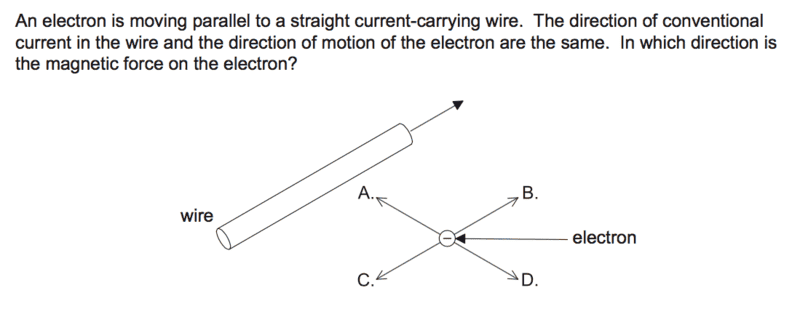# Direction of magnetic field

• Cici2017

## Homework Statement## The Attempt at a Solution

using the left hand rule, second finger points the direction of current, thumb points direction of motion and first finger the direction of magnetic field. But If direction of current and motion is the same, what do I do? Thanks~

In the LHR if C and M are parallel, there is zero force.

But you are getting a bit confused about how LHR is applied. If a conductor is in a magnetic field LHR tells you which way the force (or Motion of the conductor) will be. But the electrons, constrained within the conductor, are moving in the direction of the current (though in the opposite sense for conventional current.)

Here the (original) motion of the electrons is not the "thumb" direction : the (original) motion of the electrons is in the current direction (but opposite sense.) It is perpendicular to the field from the wire current, so you will see a force on the electron represented by the "thumb" direction (and sense.)

Maybe you should consider the electron as if it were traveling along a conductor/wire. What force would that conductor experience?

In the LHR if C and M are parallel, there is zero force.

But you are getting a bit confused about how LHR is applied. If a conductor is in a magnetic field LHR tells you which way the force (or Motion of the conductor) will be. But the electrons, constrained within the conductor, are moving in the direction of the current (though in the opposite sense for conventional current.)

Here the (original) motion of the electrons is not the "thumb" direction : the (original) motion of the electrons is in the current direction (but opposite sense.) It is perpendicular to the field from the wire current, so you will see a force on the electron represented by the "thumb" direction (and sense.)

Maybe you should consider the electron as if it were traveling along a conductor/wire. What force would that conductor experience?
If the electron is traveling along a wire, in the same direction as the existing wire. The 2 wires attract. So the force is A? but the answer is D. Sorry, can you please explain a bit more, I am not really good with these ideas.

You have to be careful, as I hinted before, about the direction of current.
In the wire shown, the current is clearly shown going in direction B. So which direction are electrons flowing in that wire?
Now the electron is also moving in direction B. So if it were an electron moving along a wire, what direction would we say the current was going?

The LHR applies to the conventional direction and sense of current flow.

You have to be careful, as I hinted before, about the direction of current.
In the wire shown, the current is clearly shown going in direction B. So which direction are electrons flowing in that wire?
Now the electron is also moving in direction B. So if it were an electron moving along a wire, what direction would we say the current was going?

The LHR applies to the conventional direction and sense of current flow.
So the conventional current is the opposite, so direction of C. So the 2 repel each other, thus force is in direction of D?

Yes.

Yes.
Thank you!

As a BTW, I always forget which is LHR and which is RHR.
I think of the SI definition of the Ampere. There is a force between parallel conductors (hence parallel currents or charge flows.)
The snag is remembering whether it is attractive or repulsive, and for that I go back to Michael Faraday with his magnetic lines of force analogy. He imagined them a bit like elastic bands trying to be as short as possible, but being exclusive, unable to cross and repelling each other sideways. Once I sketch these in, the direction of force is easy. Maybe not Physics as we know it, but it helped him to have great insights in a field that was not well understood at the time.

As a BTW, I always forget which is LHR and which is RHR.
I think of the SI definition of the Ampere. There is a force between parallel conductors (hence parallel currents or charge flows.)
The snag is remembering whether it is attractive or repulsive, and for that I go back to Michael Faraday with his magnetic lines of force analogy. He imagined them a bit like elastic bands trying to be as short as possible, but being exclusive, unable to cross and repelling each other sideways. Once I sketch these in, the direction of force is easy. Maybe not Physics as we know it, but it helped him to have great insights in a field that was not well understood at the time.
Yes, my teacher also mentioned this way of visualising it.

I see you have another Q. Have you tried sketching in the lines there?

I see you have another Q. Have you tried sketching in the lines there?
Yes, I have. And I think the force is to the right. Am I right?